#### Everything You Need in One Place

Homework problems? Exam preparation? Trying to grasp a concept or just brushing up the basics? Our extensive help & practice library have got you covered.#### Learn and Practice With Ease

Our proven video lessons ease you through problems quickly, and you get tonnes of friendly practice on questions that trip students up on tests and finals.#### Instant and Unlimited Help

Our personalized learning platform enables you to instantly find the exact walkthrough to your specific type of question. Activate unlimited help now!

0/1
##### Intros
###### Lessons
1. Properties of matrix addition overview
0/8
##### Examples
###### Lessons
1. Verifying the matrix addition properties
You are given that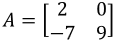and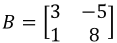and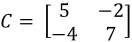. Verify the following properties:
1. Commutative property $(A+B=B+A)$
2. Associative property $(A+B)+C=A+(B+C)$
2. You are given that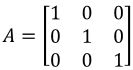and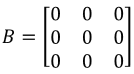and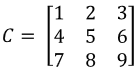. Verify the following properties:
1. Commutative property $(A+B=B+A)$
2. Associative property $(A+B)+C=A+(B+C)$
3. Let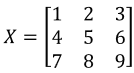. Verify the zero matrix property.
1. Let $X$ be a 2 x 2 matrix. Show that $I_n \cdot X=X$.
###### Free to Join!
StudyPug is a learning help platform covering math and science from grade 4 all the way to second year university. Our video tutorials, unlimited practice problems, and step-by-step explanations provide you or your child with all the help you need to master concepts. On top of that, it's fun - with achievements, customizable avatars, and awards to keep you motivated.
• #### Easily See Your ProgressWe track the progress you've made on a topic so you know what you've done. From the course view you can easily see what topics have what and the progress you've made on them. Fill the rings to completely master that section or mouse over the icon to see more details.
• #### Make Use of Our Learning Aids###### Practice Accuracy

See how well your practice sessions are going over time.

Stay on track with our daily recommendations.

• #### Earn Achievements as You LearnMake the most of your time as you use StudyPug to help you achieve your goals. Earn fun little badges the more you watch, practice, and use our service.
• #### Create and Customize Your AvatarPlay with our fun little avatar builder to create and customize your own avatar on StudyPug. Choose your face, eye colour, hair colour and style, and background. Unlock more options the more you use StudyPug.
###### Topic Notes
In this section, we will look at the properties of matrix addition. These properties include the dimension property for addition, commutative property, and associative property. Note that for the dimension property, we are allowed to add or subtract two matrices with the same dimensions. The commutative property states that changing the order of the addition or subtraction of two matrices lead to the same result. For the associative property, changing what matrices you add or subtract one will lead to the same answer. There are also matrix addition properties for identity and zero matrices as well. Adding a zero matrix with another matrix (call it A) will give back A. Multiplying an identity matrix with another matrix (call it B) will give back B.

During our lesson about adding and subtracting matrices we saw the way how to solve such arithmetic operations when using matrices as terms to operate. During the same lesson we introduced a few matrix addition rules to follow. The lesson of today will focus on expand about the various properties of matrix addition and their verifications.

### Addition and subtraction of matrices

To quickly summarize our concepts from past lessons let us respond to the question of how to add and subtract matrices:
We add each corresponding element on the involved matrices to produce a new matrix where such elements will occupy the same spot as their predecessors.
• How to subtract matrices?
Through exactly the same manner as we compute addition, except that we use a minus sign to operate instead of a plus sign.

Remember that as a general rule you can only add or subtract matrices which have the exact same dimensions. This comes from the fact that adding matrices with different dimensions creates an issue because not all the elements in each matrix will have a corresponding element to operate with, and so, making the operation impossible to complete.

In simple words, addition and subtraction of matrices work very similar to each other and you can actually transform an example of a matrix subtraction into an addition of matrices (more on that later). The only difference between the two operations is the arithmetic sign you use to operate: the plus sign for addition and the minus sign for subtraction.

An addition of two matrices looks as follows: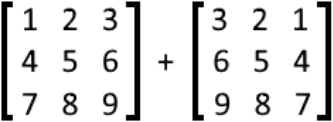Since each element will be added to its corresponding element in the other matrix. The process is simple and can be completed as shown below: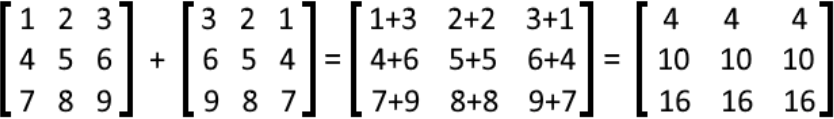Now let us look at the case in which we try to add two matrices of different order (different dimensions):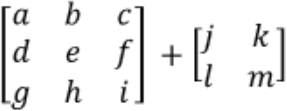For this case if we go adding element by element we will be able to add $a+j$, then $b+k$, but then you arrive to $c$ and there is not a corresponding element in the second matrix to add to $c$. The same happens if you go downwards on the first column: you would add $a+j$, $d+l$ but then the same problem comes when adding $g$, there is no correspondent element! So the whole third row and columns from the first matrix do not have a corresponding element on the second matrix since the dimensions of the matrices are not the same, and so we get to a dead end trying to find a solution for the operation. Since is no possible to resolve, we once more reaffirm the addition of two matrices of different order is undefined.

In order to talk about the properties of how to add matrices, we start by defining three examples of a constant matrix called $X$, $Y$ and $Z$, which we will use as reference.

1. $\quad$Dimension property for addition
When adding two matrices such as $X$ and $Y$, the result of this addition will be a matrix with the same dimensions of $X$ and $Y$. For example, if $X + Y$ happened to be an operation adding 2x2 matrices, then the result would be a 2x2 matrix.

Remember that adding matrices with different dimensions is not possible, a result for such operation is not defined thanks to this property, since there would be no element-by-element correspondence within the two matrices being added and thus not all of their elements would have a pair to operate with, resulting in an undefined solution. When both matrices have the same dimensions, the element-by-element correspondence is met (there is an element from each matrix to be added together which corresponds to the same place in each of the matrices), and so, a result can be obtained.

The dimension property applies in both cases, when you add or subtract matrices. Now let us describe the commutative and associative properties of matrix addition.

2. $\quad$ Commutative property
The commutative property says that when adding 2 matrices the result of the operation will not be affected if the order in which the matrices are being added is changed. Simply said, if we were to add matrices $X$ and $Y$, the condition $X + Y = Y + X$ is met.

If we have an addition of three matrices (while all of the have the same dimensions) such as $X + Y + Z$, this operation would yield the same result as if we added them in any other order, such as: $Z + Y + X = X + Z + Y = Y + Z + X$ etc.

Remember that the commutative property cannot be applied to a matrix subtraction unless you change it into an addition of matrices by applying the negative sign to the matrix that it is being subtracted. We explained this in a past lesson on how to add and subtract matrices, if you have any doubt of this just remember: The commutative property applies to matrix addition but not to matrix subtraction, unless you transform it into an addition first.

3. $\quad$ Associative property
The associative property says that when there are more than two matrices in an addition we can associate two or more of them in order to simplify the operation. In other words, if you are adding multiple matrices at the same time, you can opt for adding two of them first and then add the result to the other matrices left, in that way you would be doing multiple additions of two matrices only (as long as it needed depending on how many matrices you have) instead of adding them all at the same time (which can be complicated if there are many of them).

In simple notation, the associative property says that: $X+Y+Z = (X+Y)+Z = X+(Y+Z)$

4. $\quad$Property of the zero matrix
When adding two matrices of the same dimensions and one of them is a zero matrix, the result of the addition will be equal to the non-zero matrix. Therefore, there is always a zero matrix 0 such that $0 + X = X$ for any matrix $X$.

### Properties of matrix addition examples

The final section focuses, as always, in showing a few examples of the topics covered throughout the lesson. We will now look into matrix problems where we will add matrices in order to verify the properties of the operation.

#### Example 1

Using the three matrices given below verify the properties of matrix addition: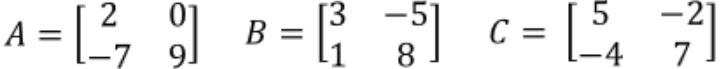a) $\quad$ In our first case we will verify the commutative property of an addition of matrices by computing the equation $A + B = B + A$

We start by computing the addition on the left hand side of the equation: $A + B$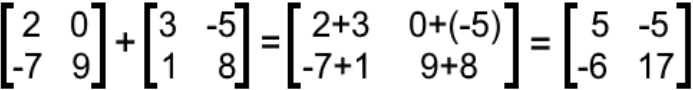Now we compute the right hand side of the equation: $B + A$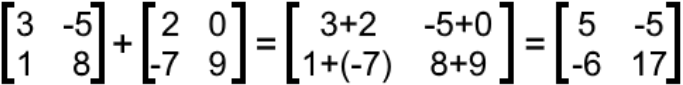As you can see, both results are the same, and thus, we have proved that the order of the matrices does not affect the result when adding them. Remember, the same does not apply to matrix subtraction, as explained in our lesson on adding and subtracting matrices.

b) $\quad$Now let us verify the associative property of matrix addition, for that we will compute the next equation containing additions of the three matrices above: $(A+B)+C = A+(B+C)$

In this case the associative property meant that whatever is found inside the parenthesis in the equations is the operation that will be performed first, Therefore, let us work through this equation first on the left hand side: $(A+B)+C$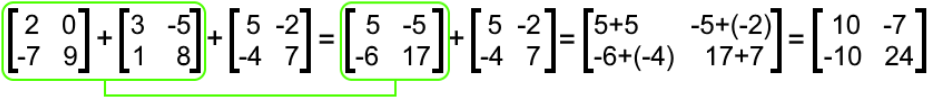Now working through the right hand side we obtain: $A+(B+C)$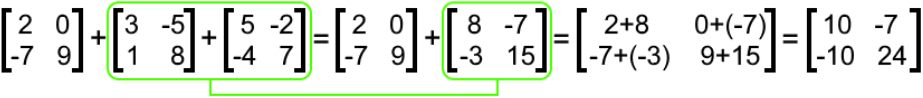As you can see, by associating matrices you are just deciding which operation to perform first, and from the case above, we know that the order in which the operations are worked through does not change the result, therefore, the same happens when you work on a whole equation by parts: picking which matrices to add first does not affect the result.

c) $\quad$We finish this exercise by verifying the dimension property for addition

In order to verify that the dimension property holds we just have to prove that when adding matrices of a certain dimension, the result will be a matrix with the same dimensions. For our given matrices $A$, $B$ and $C$, this means that since all three of them have dimensions of 2x2, when adding all three of them together at the same time the result will be a matrix with dimensions 2x2.
At this point we actually do not need to make the computation since we have already done it before in part b) of this exercise, and we have proof that when adding $A+B+C$ the resulting matrix is a 2x2 matrix, so we are done for this exercise problem.

Our website contains a video of this verification where you will notice that the only difference from that addition of $A+B+C$ shown, from the ones we have written in this lesson, is that the associative property is not being applied and the elements of all three matrices are just directly added in one step. That is usually the simplest way to add multiple matrices, just directly adding all of the corresponding elements to create the entry of the resulting matrix; still, if the addition contains way too many matrices, it is recommended that you perform the addition by associating a few of them in steps. Notice that this does not affect the final result, and so, our verification for this part of the exercise and the one in the video are equivalent to each other.

#### Example 2

Once more, we will be verifying the properties for matrix addition but now with a new set of matrices of dimensions 3x3: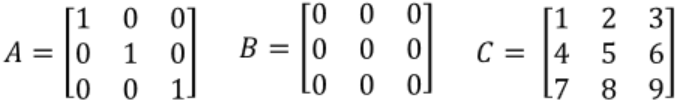a) $\quad$We start again by verifying the commutative property computing the equation $A + B = B + A$

Starting out with the left hand side of the equation: $A+B$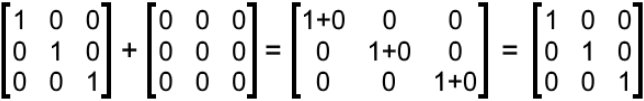Computing the right hand side of the equation: $B+A$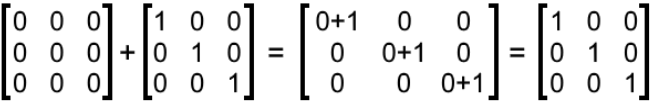And we can see the result is the same. Notice how in here we are adding a zero matrix, and so, a zero matrix does not alter the result of another matrix when added to it.

b) $\quad$To verify the associative property we compute the equation $(A+B)+C=A+(B+C)$ once more:

Since matrix $A$ is an identity matrix $I_{3}$ and matrix $B$ is a zero matrix $0_{3}$, the verification of the associative property for this case may seem repetitive; nonetheless, we recommend you to do it by hand if there are any doubts on how we obtain the next results. We start once more with the left hand side: $(A+B)+C$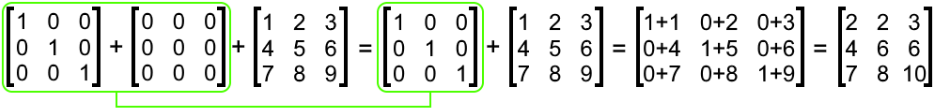Now the right hand side: $A+(B+C)$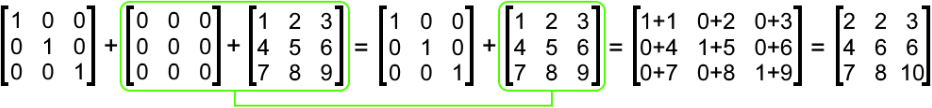c) $\quad$We finalize by verifying the dimension property for addition:

Once more, the dimension property has been already verified in part b) of this exercise, since adding all the three matrices $A+B+C$ produces a matrix which has the same dimensions as the original three: 3x3.

Notice that when adding matrix $A+B+C$ you can play around with both the commutative and the associative properties of matrix addition, and compute the calculation in different ways. So always do it as it is more convenient to you (either the simplest way you find to perform the calculation, or just a way you have a preference for), this facilitate your understanding on the topic.

#### Example 3

Verify the zero matrix property using matrix $X$ as shown below: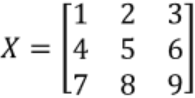Remember that the zero matrix property says that there is always a zero matrix $0$ such that $0+X=X$ for any matrix $X$. In other words, when adding a zero matrix to any matrix, as long as they have the same dimensions, the result will be equal to the non-zero matrix.
This particular case was already seen in example 2, part b). Let us write it explicitly below using matrix $X$: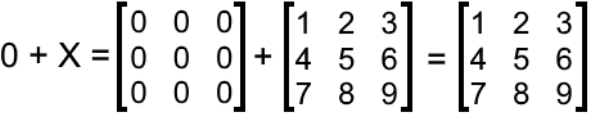#### Example 4

Let $X$ be any 2x2 matrix. If we use the identity matrix with the appropriate dimensions and multiply $X$ to it, show that $I_{n} \cdot X = X$. For this case we define $X$ as any matrix with dimensions 2x2, therefore, it doesnt matter the elements it contains inside. Defining X as shown below: nts it contains inside. Defining $X$ as shown below: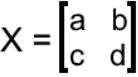And in order to perform the multiplication we know that the identity matrix will have dimensions of 2x2, and so, the multiplication goes as follows: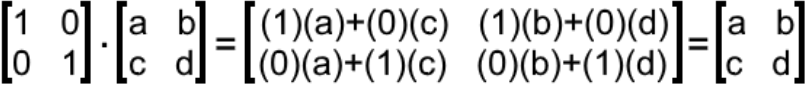This last problem has been an example of scalar multiplication of matrices, and has been included for this lesson in order to prepare you for the next one. On our next session you will see an assortment of exercises about scalar multiplication and its properties which may sometimes include adding and subtracting matrices.

$\star \star \star$

It is time to finalize our lesson for this topic, but before we go onto the next one, we would like to let you know that if you prefer an explanation of matrix addition using variable algebra notation (variables and subindexes defining the matrices) or just if you want to see a different approach at notate and resolve matrix operations, we recommend you to visit the next lesson on the properties of matrix arithmetic.

See you in the next lesson!
Let the matrices $X,Y$ and $Z$ have equal dimensions. Then we have the following matrix addition properties:

If $X$ and $Y$ has the same dimensions, then $X+Y$ also has the same dimensions.
$X+Y=Y+X$
$(X+Y)+Z=X+(Y+Z)$
There is always a zero matrix $O$ such that $O+X=X$ for any matrix $X$.
Let $X$ be a $n$ by $n$ matrix. Then there is an identity matrix $I_n$ such that $I_n \cdot X=X$.